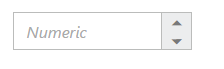# Behavior Settings

28 Jun 201813 minutes to read

## Decimal Places

The decimalPlaces property specifies number of values allowed after the decimal point.The default value of decimalPlaces property is 0. i.e., By default you cannot specify decimal value in NumericTextBox. We need to add this property to allow decimal values. To set the decimalPlaces to “-1”, that allows the decimals without any limit in NumericTextBox control.

### Configure Decimal Places

The following steps explain the implementation of decimalPlaces in NumericTextBox.

In the HTML page set the corresponding <input> elements for rendering NumericTextBox control.

• HTML
• ``<input id="numeric" type="text" />``
• JAVASCRIPT
• ``````\$("#numeric").ejNumericTextbox({
value: 333,
decimalPlaces: 3
});``````

The output for NumericTextBox with decimalPlaces is as follows.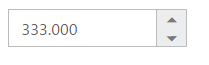## Persistence Support

The NumericTextBox widgets provides the state maintenance support. You can maintain the previous changes made in the control after a page refresh.

### Configure Persistence Support

The following steps explain the implementation of enablePersistence in NumericTextBox.

In the HTML page set the corresponding <input> elements for rendering NumericTextBox control.

• HTML
• ``<input id="numeric" type="text" />``
• JAVASCRIPT
• ``````\$("#numeric").ejNumericTextbox({
value: 11,
enablePersistence: true
});``````

The output for NumericTextBox with enablePersistence is as follows. You can change the value of NumericTextBox and reload the web page.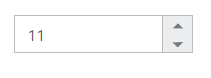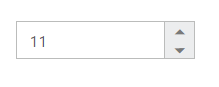NumericTextBox after changing the value and after page refresh

## Strict Mode Support

NumericTextBox allows you to use the strict mode option by setting the enableStrictMode property. You can set the minValue and maxValue to the controls to enable strict mode functionality. Default value of this property is false. When the textbox value exceeds the maxValue, it restricts the exceeded value and returns the maxValue. Likewise when the textbox value goes below minValue, it restricts the new value and returns the minValue. When this property is true, it will not restrict the specified value and an error class will be added to indicate wrong value is provided to the NumericTextBox.

### Configure Strict Mode Support

The following steps explain the implementation of enableStrictMode in NumericTextBox .

In the HTML page set the corresponding <input> elements for rendering NumericTextBox control.

• HTML
• ``<input id="numeric" type="text" />``
• JAVASCRIPT
• ``````\$("#numeric").ejNumericTextbox({
value: 10, //value(10) exceeds maxValue(5), so it will add the error class.
minValue: -3,
maxValue:5,
enableStrictMode: true
});``````

The output for NumericTextBox when enableStrictMode is “true” is as follows.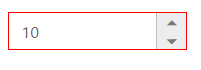## Enabled or Disabled

The NumericTextBox control has an option to enable or disable its element. You can set the enabled property as “true” to enable the NumericTextBox control.
Also you can enable/disable the NumericTextBox by using enable and disable methods.

### Configure Enabled or Disabled

The following steps explains the implementation of enabled in NumericTextBox.

In the HTML page set the corresponding <input> elements for rendering NumericTextBox control.

• HTML
• ``<input id="numeric" type="text" />``
• JAVASCRIPT
• ``````\$("#numeric").ejNumericTextbox({
value: 1,
enabled: false
});``````

The output for NumericTextBox when enabled is “false” and when enabled is “true”.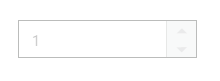NumericTextBox with enabled as false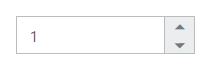NumericTextBox with enabled as true

The NumericTextBox size can be modified by using the height and width properties. You can customize the size of NumericTextBox by using these properties.

### Configure Height and Width

The following steps explain the implementation of height and width in NumericTextBox .

In the HTML page set the corresponding <input> elements for rendering NumericTextBox control.

• HTML
• ``<input id="numeric" type="text" />``
• JAVASCRIPT
• ``````\$("#numeric").ejNumericTextbox({
width: 100, height: 50,value:1
});``````

The output for NumericTextBox after setting “height” and “width” is as follows.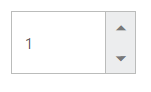## Increment Step

The incrementStep property is used to increase or decrease the amount of value in the NumericTextBox control.

### Configure Increment Step

The following steps explain the implementation of incrementStep in NumericTextBox.

In the HTML page set the corresponding <input> elements for rendering NumericTextBox control.

• HTML
• ``<input id="numeric" type="text" />``
• JAVASCRIPT
• ``````\$("#numeric").ejNumericTextbox({
value:1,
incrementStep: 2
});``````

Output of Numeric textbox with incrementStep is as follows.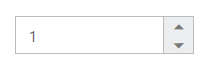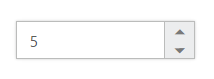NumericTextBox after increasing two step

## Define Name

When you have placed the NumericTextBox in a form, the name property is used to send the field value at form submission. The default value of the name property is null.

### Configure Name

The following steps explain the implementation of name in NumericTextBox .

In the HTML page set the corresponding <input> elements for rendering NumericTextBox control.

• HTML
• ``<input id="numeric" type="text" />``
• JAVASCRIPT
• ``````\$("#numeric").ejNumericTextbox({
value:12,
name: "numeric"
});``````

## Define Value

The value of NumericTextBox can be assigned by using the value property. The default value for value property is null.
You can get the value of NumericTextBox by using getValue method.

### Configure Value

The following steps explain the implementation of value in NumericTextBox .

In the HTML page set the corresponding <input> elements for rendering NumericTextBox control.

• HTML
• ``<input id="numeric" type="text" />``
• JAVASCRIPT
• ``````\$("#numeric").ejNumericTextbox({
value: 12
});``````

The output for NumericTextBox with the value property is as follows.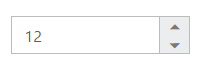## Define maxValue and minValue

### maxValue

The maximum limit value can be assigned to the NumericTextBox by using the maxValue property. The default value of maxValue property is 1.7976931348623157e+308.

### minValue

The minimum limit value can be assigned to the NumericTextBox by using the minValue property. The default value of minValue property is -1.7976931348623157e+308.

### Configure maxValue and minValue

The following steps explain the implementation of maxValue and minValue in NumericTextBox .

In the HTML page set the corresponding <input> elements for rendering NumericTextBox control.

• HTML
• ``<input id="numeric" type="text" />``
• JAVASCRIPT
• ``````//NumericTextBox with maxValue
\$("#numeric").ejNumericTextbox({
maxValue: 2,
value:3
});

//NumericTextBox with minValue
\$("#numeric").ejNumericTextbox({
minValue: -1,
value:-2
});``````

The output for NumericTextBox with basic properties is as follows.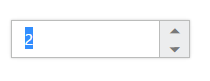NumericTextBox with maxValue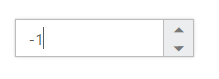NumericTextBox with minValue

The NumericTextBox supports read only option. When you enable the readOnly property to the control, the value cannot be changed in the NumericTextBox . You can set the readOnly property as “true” to enable this option.

The following steps explain the implementation of readOnly in NumericTextBox .

In the HTML page set the corresponding <input> elements for rendering NumericTextBox control.

• HTML
• ``<input id="numeric" type="text" />``
• JAVASCRIPT
• ``````\$("#numeric").ejNumericTextbox({
value: 1,
});``````

The output for NumericTextBox when readOnly is “true” is as follows. The NumericTextBox value cannot be edited or changed.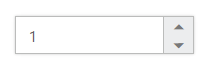## Appearance

### Theme

The NumericTextBox control style and appearance can be controlled based on CSS classes. In order to apply styles to the NumericTextBox control, you need to refer 2 files namely, ej.widgets.core.min.css and ej.theme.min.css. If the file ej.web.all.min.css is referred, then it is not necessary to include the files ej.widgets.core.min.css and ej.theme.min.css in your project, as ej.web.all.min.css is the combination of these two.

By default, there are 17 themes support available for Textbox control namely:

• bootstrap
• flat-azure
• flat-azure-dark
• fat-lime
• flat-lime-dark
• flat-saffron
• flat-saffron-dark
• high-contrast-01
• high-contrast-02
• material
• office-365

### CSS Class

The CSS can be customized by using the cssClass in the NumericTextBox . You can customize the NumericTextBox with various cssClass properties to appear like your desired control.

### Configure CSS Class

The following steps explain the implementation of cssClass in NumericTextBox .

In the HTML page set the corresponding <input> elements for rendering NumericTextBox controls.

• HTML
• ``<input id="numeric" type="text" />``
• JAVASCRIPT
• ``````\$("#numeric").ejNumericTextbox({
value: 1,
cssClass: "customCss"
});``````

Customize the CSS properties in custom CSS class.

• CSS
• ``````<style>
.customCss .e-box {
border-color: #9d241b;
}
.customCss .e-input {
background-color: #f6db8d;
}
.customCss .e-select {
background-color: #ecf6ac;
border-color: #3c36e7;
}
</style>``````

The output for NumericTextBox after applying cssClass is as follows.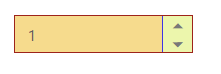## Rounded Corner Support

The NumericTextBox provides you with rounded corner support whose appearance is different from normal textbox controls.

###Configure Rounded Corner Support

The following steps explain the implementation of showRoundedCorner in NumericTextBox .

In the HTML page set the corresponding <input> elements for rendering NumericTextBox control.

• HTML
• ``<input id="numeric" type="text" />``
• JAVASCRIPT
• ``````\$("#numeric").ejNumericTextbox({
value: 1,
showRoundedCorner: true
});``````

The output for NumericTextBox when showRoundedCorner is “true”.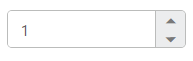## Spin Button Support

The NumericTextBox provides you the option as to whether to display the spin button in the widget or remove it from the control by using showSpinButton property.

### Configure Spin Button

The following steps explain the implementation of showSpinButton in NumericTextBox .

In the HTML page set the corresponding <input> elements for rendering NumericTextBox controls.

• HTML
• ``<input id="numeric" type="text" />``
• JAVASCRIPT
• ``````\$("#numeric").ejNumericTextbox({
value: 1,
showSpinButton: false
});``````

The output for NumericTextBox when showSpinButton is “false”.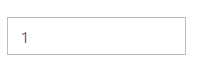## Water Mark Text Support

The NumericTextBox provide water mark text support. You can display the initial value in the control by water mark.

### Configure Water Mark Text

The following steps explain the implementation of watermarkText in NumericTextBox .

In the HTML page set the corresponding <input> elements for rendering NumericTextBox control.

• HTML
• ``<input id="numeric" type="text" />``
• JAVASCRIPT
• ``````\$("#numeric").ejNumericTextbox({
watermarkText: "Numeric"
});``````

The output for NumericTextBox after applying watermarkText is as follows.